Home Practice
For learners and parents For teachers and schools
Textbooks
Full catalogue
Pricing SupportLog in

We think you are located in United States. Is this correct?

# 5.4 Conservation of energy

## 5.4 Conservation of energy (ESCMG)

There are two categories of forces we will consider, conservative and non-conservative.

Conservative force
A conservative force is one for which work done by or against it depends only on the starting and ending points of a motion and not on the path taken.

A conservative force results in stored or potential energy and we can define a potential energy ($$E_p$$) for any conservative force.

Gravity is a conservative force and we studied gravitational potential energy in Grade 10. We now have all the concepts we need to actually deduce this ourselves. Let us consider pushing a ball up a number of different slopes.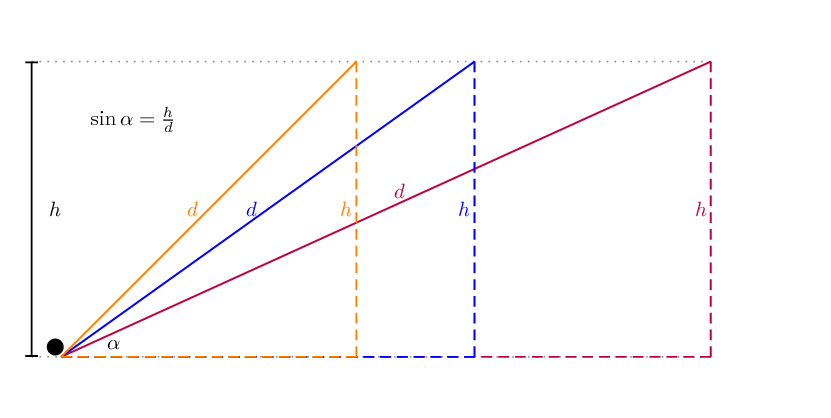Figure 5.5: Three different slopes are shown, all rising to a height of $$h$$. The imaginary right-angled triangle is shown for each slope. $$d$$ is the length of the slope. $$\alpha$$ is the angle the slope makes with the horizontal.

The slope, of length $$d$$ is the hypotenuse of an imaginary right-angled triangle. The work done by gravity while pushing a ball of mass, $$m$$, up each of the slopes can be calculated. We know that the component of the gravitational force parallel to the slope is $$\vec{F}_{gx}=\vec{F}_g\sin\alpha$$ down the slope.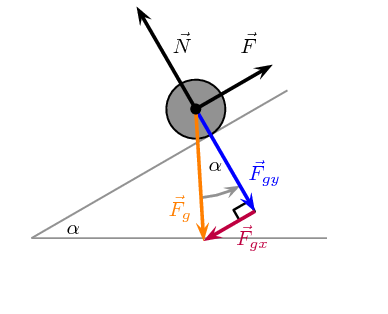The work done by gravity when the force $$\vec{F}$$ pushes the ball up the slope will be negative because the direction of the motion and $$\vec{F}_g\sin\alpha$$ are opposite.

\begin{align*} W_g &= F d \cos\theta \\ &= (\vec{F}_g\sin\alpha) d (-1) \\ &= - \vec{F}_g (\sin\alpha) d \\ &= - \vec{F}_g \left(\frac{\text{opposite}}{\text{hypotenuse}}\right) d \\ &= - \vec{F}_g \left(\frac{h}{d}\right) d \\ &= - \vec{F}_g h \end{align*}

This final result is independent of the angle of the slope. This is because $$\sin\alpha=\frac{\text{opposite}}{\text{hypotenuse}}=\frac{h}{d}$$ and so the distance cancels out. If the ball moves down the slope the only change is the sign, the work done by gravity still only depends on the change in height. This is why mechanical energy includes gravitational potential energy and is conserved. If an object goes up a distance $$h$$ gravity does negative work, if it moves back down $$h$$ gravity does positive work, but the absolute amount of work is the same so you `get it back', no matter what path you take!

This means that the work done by gravity will be same for the ball moving up any of the slopes because the end position is at the same height. The different slopes do not end in exactly the same position in the picture. If we break each slope into two sections as show in Figure 5.6 then we have 3 different paths to precisely the same end-point. In this case the total work done by gravity along each path is the sum of the work done on each piece which is just related to the height. The total work done is related to the total height.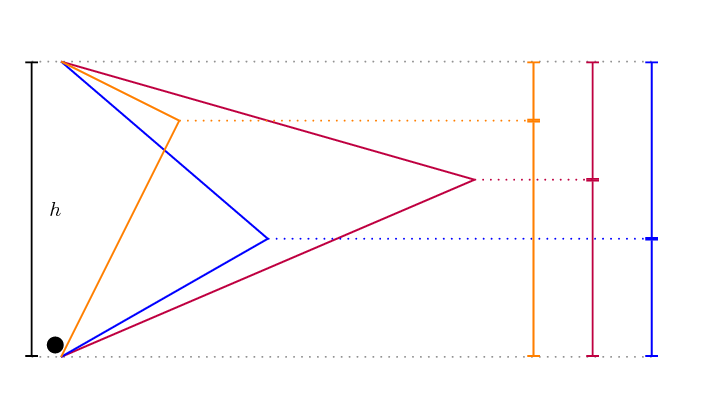Figure 5.6: Three different paths that lead from the same start-point to the same-end point. Each path leads to the same overall change in height, $$h$$, and, therefore, the same work done by gravity.

There are other examples, when you wind up a toy, an egg timer, or an old-fashioned watch, you do work against its spring and store energy in it. (We treat these springs as ideal, in that we assume there is no friction and no production of thermal energy.) This stored energy is recoverable as work, and it is useful to think of it as potential energy contained in the spring.

The total work done by a conservative force results in a change in potential energy, $$\Delta E_p$$. If the conservative force does positive work then the change in potential energy is negative. Therefore:

$W_{\text{conservative}}=-\Delta E_p$
Non-conservative force
A non-conservative force is one for which work done on the object depends on the path taken by the object.

Non-conservative forces do not imply that total energy is not conserved. Total energy is always conserved. Non-conservative forces mean that mechanical energy isn't conserved in a particular system which implies that the energy has been transferred in a process that isn't reversible.

Friction is a good example of a non-conservative force because if removes energy from the system so the amount of mechanical energy is not conserved. Non-conservative forces can also do positive work thereby increasing the total mechanical energy of the system.

The energy transferred to overcome friction depends on the distance covered and is converted to thermal energy which can't be recovered by the system.

### Non-conservative forces and work-energy theorem (ESCMH)

We know that the net work done will be the sum of the work done by all of the individual forces:

\begin{align*} F_{\text{net}} & = F_{\text{conservative}}+F_{\text{non-conservative}} \\ & (\text{the work done by these forces yields}) \\ W_{\text{net}} & = W_{\text{conservative}}+W_{\text{non-conservative}} \\ & (\text{using the work-energy theorem}) \\ \Delta E_k & = W_{\text{conservative}}+W_{\text{non-conservative}}\\ &(\text{using}~W_{\text{conservative}}=-\Delta PE) \\ \Delta E_k & = -\Delta E_p +W_{\text{non-conservative}} \\ W_{\text{non-conservative}} & = \Delta E_k + \Delta E_p \end{align*}

When the non-conservative forces oppose the motion, the work done by the non-conservative forces is negative, causing a decrease in the mechanical energy of the system. When the non-conservative forces do positive work, energy is added to the system. If the sum of the non-conservative forces is zero then mechanical energy is conserved.

## Worked example 9: Sliding footballer [credit: OpenStax College Physics]

Consider the situation shown where a football player slides to a stop on level ground. Using energy considerations, calculate the distance the $$\text{65,0}$$ $$\text{kg}$$ football player slides, given that his initial speed is $$\text{6,00}$$ $$\text{m·s^{-1}}$$ and the force of friction against him is a constant $$\text{450}$$ $$\text{N}$$.### Analyse the problem and determine what is given

Friction stops the player by converting his kinetic energy into other forms, including thermal energy. In terms of the work-energy theorem, the work done by friction, which is negative, is added to the initial kinetic energy to reduce it to zero. The work done by friction is negative, because $$F_f$$ is in the opposite direction of the motion (that is, $$θ=\text{180}\text{ º}$$ , and so $$\cos\theta=-1$$). Thus $$W_{\text{non-conservative}} = -F_f \Delta x$$.

There is no change in potential energy.

### Next we calculate the distance using the conservation of energy

We begin with conservation of energy:

$W_{\text{non-conservative}} = \Delta EK + \Delta PE$

The equation expands to:

\begin{align*} W_{\text{non-conservative}} & = \Delta E_k + \Delta E_p \\ & = E_{k,f} - E_{k,i} + (0) \\ -F_f \Delta x & = E_{k,f} - E_{k,i} \\ -F_f \Delta x & = (0) - E_{k,i} \\ F_f \Delta x & = \frac{1}{2}mv_i^2 \\ \Delta x &= \frac{mv_i^2}{2F_f} \\ & = \frac{(\text{65,0})(\text{6,00})^2}{2(\text{450})} \\ & = \text{2,60}\text{ m} \end{align*}

The footballer comes to a stop after sliding for $$\text{2,60}$$ $$\text{m}$$.

Discussion

The most important point of this example is that the amount of non-conservative work equals the change in mechanical energy. For example, you must work harder to stop a truck, with its large mechanical energy, than to stop a mosquito.

## Worked example 10: Sliding up a slope [credit: OpenStax College Physics]

The same $$\text{65,0}$$ $$\text{kg}$$ footballer running at the same speed of $$\text{6,00}$$ $$\text{m·s^{-1}}$$ dives up the inclined embankment at the side of the field. The force of friction is still $$\text{450}$$ $$\text{N}$$ as it is the same surface, but the surface is inclined at $$\text{5}$$ $$\text{º}$$. How far does he slide now?

### Analyse the question

Friction stops the player by converting his kinetic energy into other forms, including thermal energy, just in the previous worked example. The difference in this case is that the height of the player will change which means a non-zero change to gravitational potential energy.

The work done by friction is negative, because $$F_f$$ is in the opposite direction of the motion (that is, $$θ=\text{180}\text{ º}$$).

We sketch the situation showing that the footballer slides a distance $$d$$ up the slope.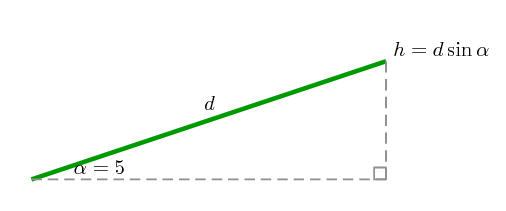In this case, the work done by the non-conservative friction force on the player reduces the mechanical energy he has from his kinetic energy at zero height, to the final mechanical energy he has by moving through distance $$d$$ to reach height $$h$$ along the incline. This is expressed by the equation:

\begin{align*} W_{\text{non-conservative}} & = \Delta E_k + \Delta E_p \\ & = E_{k,f} - E_{k,i} + E_{p,f} - E_{p,i} \\ W_{\text{non-conservative}} + E_{k,i} + E_{p,i} & = E_{k,f} + E_{p,f} \end{align*}

We know that:

• the work done by friction is $$W_{\text{non-conservative}} = - F_f d$$,
• the initial potential energy is $$E_{p,i} = mg(0)=0$$,
• the initial kinetic energy is $$E_{k,i}=\frac{1}{2}mv_i^2$$,
• the final kinetic energy is $$E_{k,f}=0$$, and
• the final potential energy is $$E_{p,f}=mgh=mgd \sin \theta$$.

### Solve for the distance

\begin{align*} W_{\text{non-conservative}} + E_{k,i} + E_{p,i} & = E_{k,f} + E_{p,f} \\ (-F_fd) + \frac{1}{2}mv_i^2 + 0 & = 0 +mgd\sin \theta \\ (-F_fd) - mgd\sin \theta &=-\frac{1}{2}mv_i^2 \\ d (F_f + mg\sin\theta) & = \frac{1}{2}mv_i^2 \\ d & = \frac{\frac{1}{2}mv_i^2}{F_f + mg\sin\theta} \\ & = \frac{\frac{1}{2}(\text{65,0})(\text{6,00})^2}{\text{450}+(\text{65,0})(\text{9,8})\sin(\text{5,00})}\\ & = \text{2,31}\text{ m} \end{align*}

The player slides for $$\text{2,31}$$ $$\text{m}$$ before stopping.

As might have been expected, the footballer slides a shorter distance by sliding uphill. Note that the problem could also have been solved in terms of the forces directly and the work energy theorem, instead of using the potential energy. This method would have required combining the normal force and force of gravity vectors, which no longer cancel each other because they point in different directions, and friction, to find the net force. You could then use the net force and the net work to find the distance $$d$$ that reduces the kinetic energy to zero. By applying conservation of energy and using the potential energy instead, we need only consider the gravitational potential energy, without combining and resolving force vectors. This simplifies the solution considerably.

## Energy conservation

Textbook Exercise 5.3

A $$\text{60,0}$$ $$\text{kg}$$ skier with an initial speed of $$\text{12,0}$$ $$\text{m·s^{-1}}$$ coasts up a $$\text{2,50}$$ $$\text{m}$$-high rise as shown in the figure. Find her final speed at the top, given that the coefficient of friction between her skis and the snow is $$\text{0,0800}$$. (Hint: Find the distance traveled up the incline assuming a straight-line path as shown in the figure.)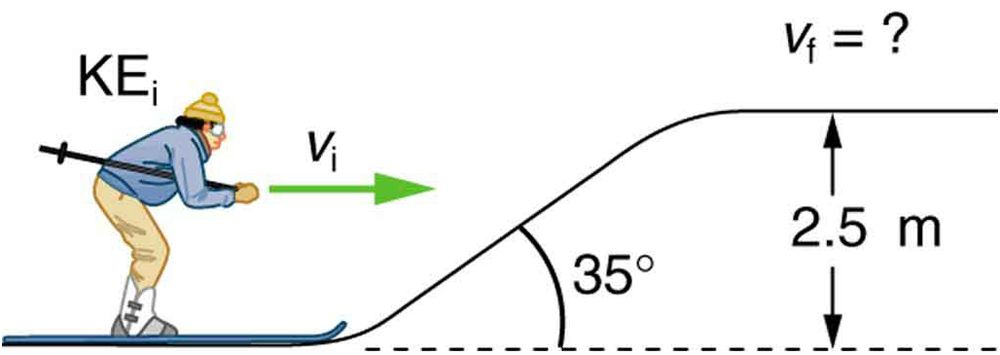We need to determine the length of the slope as this is the distance over which friction acts as well as the normal force of the skier on the slope to determine the magnitude of the force due to friction. The normal force balances the component of gravity perpendicular to the slope, therefore: \begin{align*} F_{\text{friction}}&= - \mu N \\ &= - \mu F_g\cos\theta \\ &= - \mu mg \cos\theta \end{align*} The length of the slope will be $$\Delta x = \dfrac{h}{\sin\theta}$$.

\begin{align*} W_{\text{non-conservative}} + E_{k,i} + E_{p,i} & = E_{k,f} + E_{p,f} \\ -\mu mg\cos\theta \dfrac{h}{\sin\theta} + \frac{1}{2}mv_i^2 +mgh_i &= \frac{1}{2}mv_f^2 + mgh_f \\ -\mu g\cos\theta \dfrac{h}{\sin\theta} + \frac{1}{2}v_i^2 +gh_i &= \frac{1}{2}v_f^2 + gh_f \\ \frac{1}{2}v_f^2 + gh_f &= -\mu g\cos\theta \dfrac{h}{\sin\theta} + \frac{1}{2}v_i^2 +gh_i \\ \frac{1}{2}v_f^2 + &= -\mu g\cos\theta \dfrac{h}{\sin\theta} + \frac{1}{2}v_i^2 +g(h_i -h_f) \\ v_f^2 &= -2\mu g\cos\theta \dfrac{h}{\sin\theta} + v_i^2 +2g(h_i -h_f) \end{align*} \begin{align*} v_f^2 &= -2(0,08)(9,8) \cos(35) \dfrac{2.5}{\sin(35)} + (12)^2 +2(9,8)(-2,5) \\ v_f & = \sqrt{89,4016598} \\ v_f & = \text{9,46}\text{ m·s$^{-1}$} \end{align*} $$\text{9,46}$$ $$\text{m·s^{-1}}$$
How high a hill can a car coast up (engine disengaged) if work done by friction is negligible and its initial speed is $$\text{110}$$ $$\text{km·h^{-1}}$$?

\begin{align*} 110 km/hr&= 110 \times \frac{1000}{3600} \\ &= \text{30,56}\text{ m·s$^{-1}$} \end{align*} \begin{align*} mgh&= \frac{1}{2}mv^2 \\ h&= \frac{v^2}{2g} \\ &= \frac{(\text{30,56})^2}{2(\text{9,8})} \\ &= \text{47,65}\text{ m} \end{align*}

$$\text{47,65}$$ $$\text{m}$$
If, in actuality, a $$\text{750}$$ $$\text{kg}$$ car with an initial speed of $$\text{110}$$ $$\text{km·h^{-1}}$$ is observed to coast up a hill to a height $$\text{22,0}$$ $$\text{m}$$ above its starting point, how much thermal energy was generated by friction?

Kinetic energy was converted into potential energy. The addition of friction as a dissipative force ensures that some of the kinetic energy is lost as thermal energy. The difference in the potential energy gained with and without friction is the energy lost to friction. The gravitational potential energy gained is only related to height so the difference in height allows us to determine the energy lost to friction quickly.

Without friction the car rose to a height of $$\text{47,65}$$$$\text{m}$$, with friction the height was only $$\text{22}$$$$\text{m}$$. The energy lost to friction is equivalent to the energy required to increase the gravitational potential energy by raising the vehicle a height of $$\text{25,65}$$$$\text{m}$$. Therefore the energy lost to friction is:

\begin{align*} W&= mgh \\ &= (750)(9,8)(25.65) \\ & = \text{188 527,5}\text{ J} \end{align*}

We calculated the gravitational potential energy that was lost as friction but the correct answer will be negative this quantity as the work done by friction is negative: -$$\text{188 527,5}$$ $$\text{J}$$

What is the average force of friction if the hill has a slope $$\text{2,5}$$ $$\text{º}$$ above the horizontal?

We will assume a constant force of friction. The disance over which the friction acted is the length of the slope, we know the angle of the slope and the vertical height so we can calculate the distance (hypotenuse). We can use the definition of work to calculate the force, remember that friction acts opposite to the displacement:

\begin{align*} W_{friction}&= F_{friction}\Delta x \cos\theta \\ &= -F \Delta x \\ &= -F \frac{h}{\sin(2.5)} \\ -\text{188 527,5}&= -F \frac{h}{\sin(2.5)} \\ F&= \text{188 527,5} \frac{\sin(2.5)}{22} \\ F&= \text{373,79}\text{ N} \end{align*} $$\text{373,79}$$ $$\text{N}$$
A bullet traveling at 100 m/s just pierces a wooden plank of 5 m. What should be the speed (in m/s) of the bullet to pierce a wooden plank of same material, but having a thickness of 10m?

Final speed and hence final kinetic energy are zero in both cases. From "work- kinetic energy" theorem, initial kinetic energy is equal to work done by the force resisting the motion of bullet. As the material is same, the resisting force is same in either case. If subscript "1" and "2" denote the two cases respectively, then:

5m:

\begin{align*} 0−\frac{1}{2}mv_1^2 & = −Fx_1 \\ \frac{1}{2}m(100)^2=F\times 5 \end{align*}

10m:

\begin{align*} 0−\frac{1}{2}mv_2^2 & = −Fx_2 \\ \frac{1}{2}mv_2^2=F\times 10 \end{align*}

Take the ratio of these two equations:

\begin{align*} \frac{v_2^2}{(100)^2} &=\frac{F\times 10}{F\times 5}=2 \\ v_2^2 & = 2 \times 10000 = 20000 \\ v_2 & = \text{141,4}\text{ m·s$^{-1}$} \end{align*}

$$\text{141,4}$$ $$\text{m·s^{-1}}$$